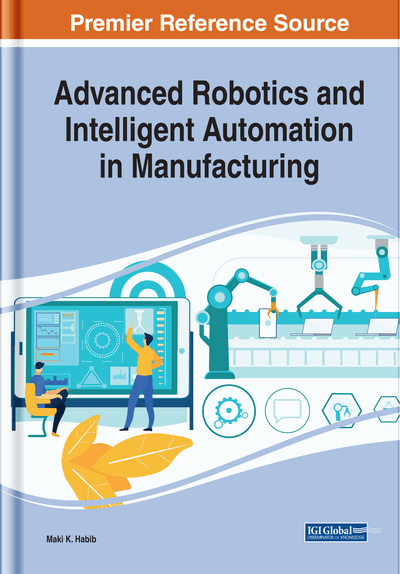# An Efficient Learning of Neural Networks to Acquire Inverse Kinematics Model

Fusaomi Nagata, Maki K. Habib, Keigo Watanabe
DOI: 10.4018/978-1-7998-1382-8.ch008
OnDemand:
(Individual Chapters)
Available
\$37.50
No Current Special Offers

## Abstract

In this chapter, effective learning approach of inverse kinematics using neural networks with efficient weights update ability has been presented for a serial link structure and industrial robot. Generally, in making neural networks learn a relation among multi inputs and outputs, a desired training data set prepared in advance is used. The training data set consists of multiple pairs of input and output vectors. The input layer receives each input vector for forward computation, and it is compared with the yielded vector from the output layer. The time required for the learning process of the neural networks depends on the number of total weights in the neural networks and that of the input-output pairs in the training data set.
Chapter Preview
Top

## Introduction

Since the planning and the control of a serial link structure and of industrial robots are directly affected by the method used to solve its inverse kinematic problem, it is important and an essential requirement for such structures to have the capability to develop a highly accurate and efficient solutions to the inverse kinematic problem. The inverse kinematics problem is defined as how to map a task coordinate system from Cartesian space to joint space given desired task space information for trajectory tracking (Xu et al, 2017; Carlos et al (2018); Zhou et al, 2018).

The authors have the experience of designing and implementing a neural network based technique with the industrial robot PUMA560. The trained neural network could acquire the desired relation between the contact force with an object and the velocity (Nagata & Watanabe, 2002). This chapter introduces the design of a neural network based approach with efficient learning algorithm to solve the inverse kinematics problem of a serial link structure.

For example, when designing a leg module with a serial link structure as shown in Fig. 1 for a multi-legged robot used in mechatronics education, first of all, its inverse kinematics problem must be solved. Up to now, biomimetic artificial neural network approaches have been devoted to robotic inverse kinematics problems. The neural network technique mimicking the human grain is known as one of the important results in biomedical engineering. In this first section, several related researches that challenged to solve inverse kinematics problems of manipulators or mechatronics systems are surveyed. Mao and Hsia proposed a neural network approach to solve the inverse kinematics of redundant robot manipulators in an environment with obstacles (Mao & Hsia, 1997), in which only the knowledge of the robot forward kinematics functions was required and the neural network was trained in the inverse modeling manner. Tejomurtula and Kak (1999) proposed a solution of inverse kinematics concerning a simple two link manipulator. It was reported that the proposed neural network method yielded multiple and precise solutions suitable for real-time applications. Xia and Wang proposed a new recurrent neural network called the dual network (Xia & Wang, 2001). It was shown that the dual network was capable of asymptotic tracking for the motion control of kinematically redundant manipulators.

Koker et al. (2004) proposed a neural network based inverse kinematics solution for a three joints manipulator, in which the designed neural networks gave reliable three angles according to a given position in Cartesian coordinates. Then, neural networks and genetic algorithms were fused together to solve the inverse kinematics problem of a Stanford robotic manipulator with six-DOFs while minimize the error at the end effector (Koker, 2013). Hoang et al. proposed a differential kinematics algorithm to generate omnidirectional walking trajectory of a leg based on back-stepping control using Lyapunov stability. Simulation results for walking motion of one leg of the 6LR were shown to prove the effectiveness and applicability of the proposed controller (Hoang et al, 2014). Aggarwal et al. presented a non-conventional technique for solving the inverse kinematics problem using artificial neural networks. A feed forward multi-layer neural network was applied to this research. The inverse kinematic solution for a PUMA 560 robot was developed by training the neural network with the end-effector’s Cartesian co-ordinates and its corresponding joint angles.

## Key Terms in this Chapter

Industrial Robots: Have progressed remarkably as one of the powerful automation machines and been applied to many tasks such as welding, paining, handling, assembling and so on. General industrial robots have a serial link structure with five, six or seven DOFs pursuing the function of a human arm, so that more flexible motion than NC machine tools can be realized.

Weights Update Method: Multi-layered neural networks have to be trained to acquire the non-linear relation between input and output teaching signals. The training means weights update process to enable the neural networks to produce the output teaching signals according to the input teaching signals. In this chapter, a novel efficient weights update method is proposed.

Kinematics: Quantitatively handles the relation between joint angles in joint coordinate system and the corresponding position/orientation elements of the arm tip in Cartesian coordinate system, without considering the masses of those parts nor the torques and forces that drive the joints.

Neural Networks: Are typically composed of multiple layers and the signal traverses from the input layer to the output layer of neurons. Trained neural networks can approximate an arbitrary nonlinear function.

Serial Link Structure: Is the typical configuration of articulated-type industrial robots. Each link of the industrial robot is connected with a servo motor-driven joint for rotational motion. Flexible position and orientation motion with multi-DOFs is characterized.

Back propagation Algorithm: Is the most famous and reliable algorithm based on the concept of the steepest decent method and can train weights between each neuron in neural networks based on errors. The errors are defined as the quantity between the output of neural network and teaching signal prepared in advance.

## Complete Chapter List

Search this Book:
Reset